## Photons

The quantization of the electromagnetic field is an central result in physics. It provides a unified picture of the wave nature of light and the particle nature of light. Many triumphs of physics are revisted during the discussion such as the unification of the laws for electricity and magnetism (described by Maxwell's equations) and the law that describes wave propagation (the wave equation), the quantization of the harmonic oscillator, and Planck's radiation law. The derivation also serves as a template for the quantization of other fields. Many of the arguments presented here can be applied to phonons, magnons, plasmons, electrons, spinons, holons and other quantum particles observed in solids.

Review of Maxwell's unification of electromagnetism and optics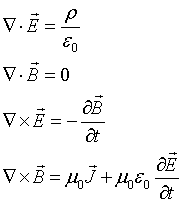The electric and magnetic fields can be expressed in terms of the vector potential A and the scalar potential V.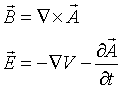We consider electromagnetic waves confined to a cavity. It is convenient to choose a cubic cavity where the length of the sides of the cavity is L. are propagating through vacuum. The source terms vanish and the electrostic potential is a constant that can be taken to be zero, ρ = 0, J = 0, V = 0. Expressed in terms of the vector potential, Maxwell's equations for this case are,It is convenient to work in Coulomb gauge where ∇·A = 0. The first three of Maxwell's equations reduce to identitites. Using the relation,the fourth equation takes the form of the wave equation,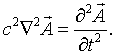Here we have used the fact that c² = 1/(μ0ε0). Solutions to the wave equation have the form,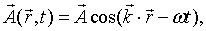where the relationship between ω and k is,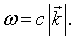The condition ∇·A = 0 requires the vector potential to be perpendicular to the propagation direction, k·A = 0.

For instance, for a wave propagating in the x-direction, the vector potential has the form,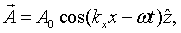The electric and magnetic fields are both perpendicular to the propagation direction in this case,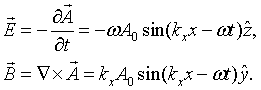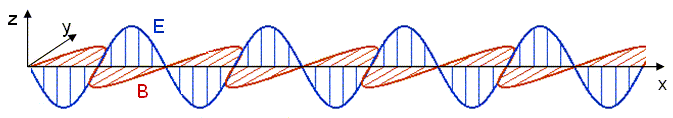Quantization

Usually a system is quantized by looking for solutions to the Schrödinger equation. Unfortunately the kinetic energy term in the Schrödinger equation includes a factor of 1/m where m is the mass of the particle. The mass of a photon is zero so this form of the Schrödinger equation is not suitable for the quantization of light. It will be necessary to start from the Lagrangian for the classical motion and use the general rules for quantization.

It is convenient to expand the waves in normal modes. Consider a cube of length L on a side with periodic boundary conditions. The spatial part of the electric field then has the form,Consider a single mode specified by the wave numbers kx, ky, and kz and the polarization. For notational convenience, we will assign a unique integer s to each mode. Substituting this mode into the wave equation results in the following equation for mode s,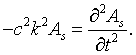The choice of the polarization plus the fact that the vector potential must be perpendicular to the propagation vector k restricts the vector potential to point in a specific direction. The Lagrangian for this mode can be found 'by inspection.' This means that we guess a Lagrangian and substitute it in the Euler-Lagrange equations. If this process yield the classical equations we are looking for, we have found the Lagrangian. The Euler-Lagrange equation for generalized coordinate q is,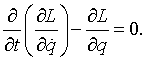In this case, the Lagrangian is,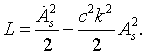The conjugate variable to As is,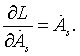The Hamiltonian is found by performing a Legrendre transformation,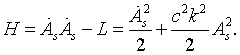To make the transition to quantum mechanics we replace the conjugate variable to As by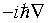in the equation Hψ = Eψ. The resulting equation that must be solved is,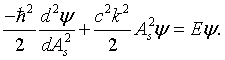This equation is mathematically equivalent to a one-dimensional harmonic oscillator. We can immediately write down the solutions. The allowed quantum energy levels are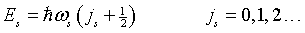where,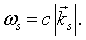The integer j represents the number of photons in mode s. The relationship between ω and k is called the dispersion relation. For electromagnetic waves in vacuum, the dispersion relation is linear and the slope of the dispersion relation is the speed of light. When light propagates through matter, we will see that the dispersion relation becomes nonlinear due to the diffration of light from the crystal lattice.

We can determine how many modes there are at every frequency if we know how many modes there are for every wavenumber k. When periodic boundary conditions are applied to a cube of length L, an integer number of wavelengths must fit in the cube, λ = L/n, where n is an integer. This restricts the allowed wavevectors to kx, ky, kz = 2πn/L. These allowed values of k form a cubic lattice in k space. The k space volume associated with each point is (2π/L)³.There are twice as many linearly independent normal modes as there are points in k-space because the electric field has two independent components that are perpendicular to the propagation direction. The number of allowed points with k values between k and k + dk is,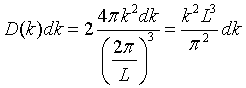Using the relationship ω = c|k|, the density of modes per unit volume in terms of frequency is,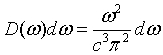In the section on the thermodynamics of non-interacting bosons, an expression for the Helmholtz free energy was derived. Since the number of photons is not conserved, the chemical potential for a photon gas is zero and the Helmholtz free energy is,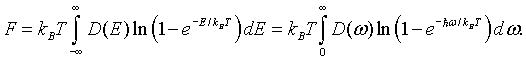Make a change of variables, x = (h/2π)ω/kBT and integrate by parts.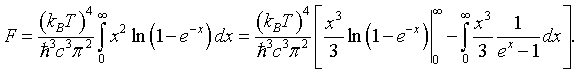The first term vanishes.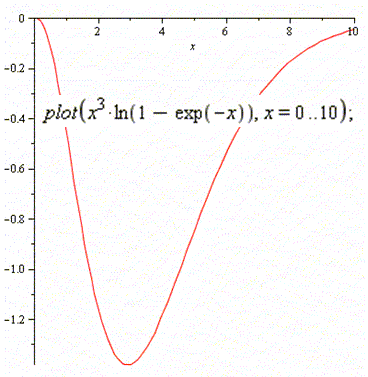The free energy is,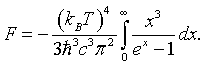This is a form of Planck's famous formula for the energy density of a blackbody. We will have to make a few manipulations to put it in a more conventional form.

The entropy is,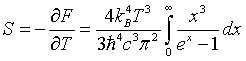The internal energy is,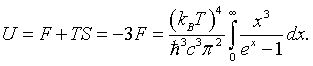The conventional form for Planck's radiation law is the internal energy density as a function of wavelength. Substituting x = hckBT yields the energy density in the appropriate form. The energy density between λ and λ + dλ is,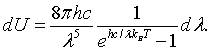u(λ) [J m-4] λ [μm]
 Temperature: T = [K]

This plot was generated for an object with a temperture of 5700 K. That is the temperture of the surface of the sun. The peak in the spectrum is then in the visible at about 0.5 μm (green). The form above can be used to generate Planck curves for other temperatures. The wavelength at the peak of the distribution can be found by differentiating the expression for the energy density and setting the result equal to zero. The result is known as Wien's law.

λmaxT = 0.0028977 m K

This is used, for instance, to determine the temperature of distant stars.

The definite integral in the expression for the internal energy and the Helmholtz free energy can be evaluated to be,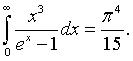The free energy is,The entropy is,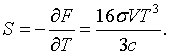The internal energy is,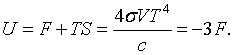This T4 relationship is known as the Stefan-Boltzmann law. The intensity radiated by a black body is,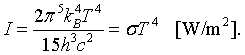The Stefan-Boltzmann constant is σ = 5.67 × 10-8 W/(m2 K4).

The pressure is,The specific heat is,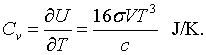Recipe for the quantization of fields

• Determine the classical normal modes.
• Quantize the normal modes.
• Knowing the all of the quantum states and corresponding eigenenergies, construct the thermodynamic partition function.
• From the partition function, deduce thermodynamic quantities.

A good discussion of non-interacting bosons from a statistical physics viewpoint can be found in Landau and Lifshitz, Statistical Physics, Part 1, and Goldstein, States of Matter.